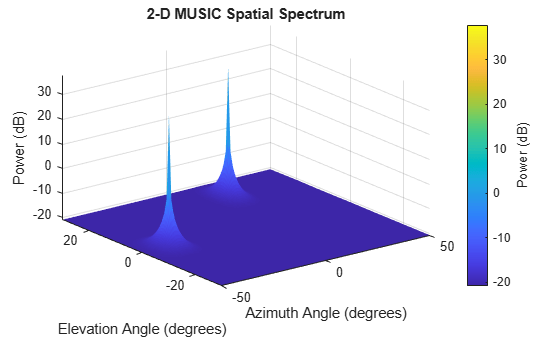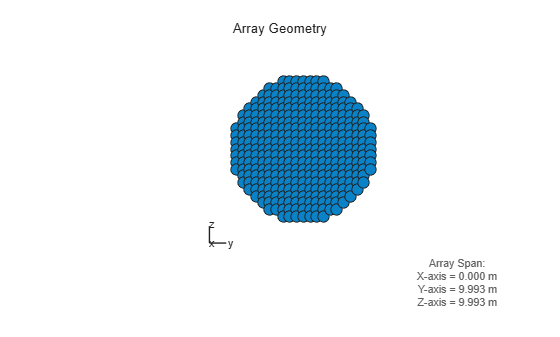# step

System object: phased.MUSICEstimator2D
Package: phased

Estimate direction of arrival using 2-D MUSIC

## Syntax

```spectrum = step(estimator,X) [spectrum,doa] = step(estimator,X) ```

## Description

Note

Instead of using the `step` method to perform the operation defined by the System object™, you can call the object with arguments, as if it were a function. For example, ```y = step(obj,x)``` and `y = obj(x)` perform equivalent operations.

`spectrum = step(estimator,X)` returns the 2-D MUSIC spectrum of a signal specified in `X`.

```[spectrum,doa] = step(estimator,X)``` also returns the signal directions of arrival angles, `doa`. To use this syntax, set the `DOAOutputPort` property to `true`.

Note

The object performs an initialization the first time the object is executed. This initialization locks nontunable properties and input specifications, such as dimensions, complexity, and data type of the input data. If you change a nontunable property or an input specification, the System object issues an error. To change nontunable properties or inputs, you must first call the `release` method to unlock the object.

## Input Arguments

expand all

2-D MUSIC estimator, specified as a `phased.MUSICEstimator2D` System object.

Received signal, specified as an M-by-N complex-valued matrix. The quantity M is the number of sample values (snapshots) contained in the signal and N is the number of sensor elements in the array.

The size of the first dimension of the input matrix can vary to simulate a changing signal length. A size change can occur, for example, in the case of a pulse waveform with variable pulse repetition frequency.

Example: `[[0;1;2;3;4;3;2;1;0],[1;2;3;4;3;2;1;0;0]]`

Data Types: `single` | `double`
Complex Number Support: Yes

## Output Arguments

expand all

2-D MUSIC spatial spectrum, returned as a nonnegative, real-valued K-length column vector representing the magnitude of the estimated MUSIC spatial spectrum. Each entry corresponds to an angle specified by the `AzimuthScanAngles` and `ElevationScanAngles` properties.

Directions of arrival of the signals, returned as a real-valued 2-by-L matrix. The direction of arrival angle is defined by the azimuth and elevation angles of the source with respect to the array local coordinate system. The first row of the matrix contains the azimuth angles and the second row contains the elevation angles. Angle units are in degrees. L is the number of signals specified by the `NumSignals` property or derived using the method specified by the `NumSignalsMethod` property.

#### Dependencies

To enable this output argument, set the `DOAOutputPort` property to `true`.

## Examples

expand all

Assume that two sinusoidal waves of frequencies 450 Hz and 600 Hz strike a URA from two different directions. Signals arrive from -37° azimuth, 0° elevation and 17° azimuth, 20° elevation. Use 2-D MUSIC to estimate the directions of arrival of the two signals. The array operating frequency is 150 MHz and the signal sampling frequency is 8 kHz.

```f1 = 450.0; f2 = 600.0; doa1 = [-37;0]; doa2 = [17;20]; fc = 150e6; c = physconst('LightSpeed'); lam = c/fc; fs = 8000;```

Create the URA with default isotropic elements. Set the frequency response range of the elements.

```array = phased.URA('Size',[11 11],'ElementSpacing',[lam/2 lam/2]); array.Element.FrequencyRange = [50.0e6 500.0e6];```

Create the two signals and add random noise.

```t = (0:1/fs:1).'; x1 = cos(2*pi*t*f1); x2 = cos(2*pi*t*f2); x = collectPlaneWave(array,[x1 x2],[doa1,doa2],fc); noise = 0.1*(randn(size(x))+1i*randn(size(x)));```

Create and execute the 2-D MUSIC estimator to find the directions of arrival.

```estimator = phased.MUSICEstimator2D('SensorArray',array,... 'OperatingFrequency',fc,... 'NumSignalsSource','Property',... 'DOAOutputPort',true,'NumSignals',2,... 'AzimuthScanAngles',-50:.5:50,... 'ElevationScanAngles',-30:.5:30); [~,doas] = estimator(x + noise)```
```doas = 2×2 -37 17 0 20 ```

The estimated DOAs exactly match the true DOAs.

Plot the 2-D spatial spectrum.

`plotSpectrum(estimator);`Assume that two sinusoidal waves of frequencies 1.6 kHz and 1.8 kHz strike a disk array from two different directions. The spacing between elements of the disk is 1/2 wavelength. Signals arrive from -31° azimuth, -11° elevation and 35° azimuth, 55° elevation. Use 2-D MUSIC to estimate the directions of arrival of the two signals. The array operating frequency is 300 MHz and the signal sampling frequency is 8 kHz.

Note: This example runs only in R2016b or later. If you are using an earlier release, replace each call to the function with the equivalent `step` syntax. For example, replace `myObject(x)` with `step(myObject,x)`.

```f1 = 1.6e3; f2 = 1.8e3; doa1 = [-31;-11]; doa2 = [35;55]; fc = 300e6; c = physconst('LightSpeed'); lam = c/fc; fs = 8.0e3;```

Create a conformal array with default isotropic elements. First, create a URA to get the element positions.

```uraarray = phased.URA('Size',[21 21],'ElementSpacing',[lam/2 lam/2]); pos = getElementPosition(uraarray);```

Extract a subset of these to form an inscribed disk.

```radius = 10.5*lam/2; pos(:,sum(pos.^2) > radius^2) = [];```

Then, create the conformal array using these positions.

```confarray = phased.ConformalArray('ElementPosition',pos); viewArray(confarray)```Set the frequency response range of the elements.

`confarray.Element.FrequencyRange = [50.0e6 600.0e6];`

Create the two signals and add random noise.

```t = (0:1/fs:1.5).'; x1 = cos(2*pi*t*f1); x2 = cos(2*pi*t*f2); x = collectPlaneWave(confarray,[x1 x2],[doa1,doa2],fc); noise = 0.1*(randn(size(x)) + 1i*randn(size(x)));```

Create and execute the 2-D MUSIC estimator to find the directions of arrival.

```estimator = phased.MUSICEstimator2D('SensorArray',confarray,... 'OperatingFrequency',fc,... 'NumSignalsSource','Property',... 'DOAOutputPort',true,'NumSignals',2,... 'AzimuthScanAngles',-60:.1:60,... 'ElevationScanAngles',-60:.1:60); [~,doas] = estimator(x + noise)```
```doas = 2×2 35 -31 55 -11 ```

The estimated DOAs exactly match the true DOAs.

Plot the 2-D spatial spectrum.

`plotSpectrum(estimator);`## Version History

Introduced in R2016b